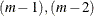### Controlling the Number of Iterations

Several options in the PROC PRINQUAL statement control the number of iterations performed. Iteration terminates when any one of the following conditions is satisfied:

• The number of iterations equals the value of the MAXITER= option.

• The average absolute change in variable scores from one iteration to the next is less than the value of the CONVERGE= option.

• The criterion change is less than the value of the CCONVERGE= option.

With the MTV method, the change in the proportion of variance criterion can become negative when the data have converged so that it is numerically impossible, within machine precision, to increase the criterion. Because the MTV algorithm is convergent, a negative criterion change is the result of very small amounts of rounding error. The MGV method displays the average squared multiple correlation (which is not the criterion being optimized), so the criterion change can become negative well before convergence. The MAC method criterion (average correlation) is never computed, so the CCONVERGE= option is ignored for METHOD=MAC. You can specify a negative value for either convergence option if you want to define convergence only in terms of the other convergence option.

With the MGV method, iterations minimize the generalized variance (determinant), but the generalized variance is not reported for two reasons. First, in most data sets, the generalized variance is almost always near zero (or will be after one or two iterations), which is its minimum. This does not mean that iteration is complete; it simply means that at least one multiple correlation is at or near one. The algorithm continues minimizing the determinant indimensions, and so on. Because the generalized variance is almost always near zero, it does not provide a good indication of how the iterations are progressing. The mean R square provides a better indication of convergence. The second reason for not reporting the generalized variance is that almost no additional time is required to compute R square values for each step. This is because the error sum of squares is a byproduct of the algorithm at each step. Computing the determinant at the end of each iteration adds more computations to an already computationally intensive algorithm.

You can increase the number of iterations to ensure convergence by increasing the value of the MAXITER= option and decreasing the value of the CONVERGE= option. Because the average absolute change in standardized variable scores seldom decreases below 1E–11, you typically do not specify a value for the CONVERGE= option less than 1E–8 or 1E–10. Most of the data changes occur during the first few iterations, but the data can still change after 50 or even 100 iterations. You can try different combinations of values for the CONVERGE= and MAXITER= options to ensure convergence without extreme overiteration. If the data do not converge with the default specifications, specify the REITERATE option, or try CONVERGE=1E–8 and MAXITER=50, or CONVERGE=1E–10 and MAXITER=200.